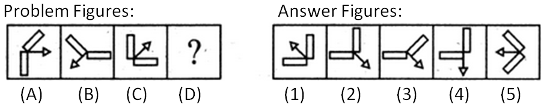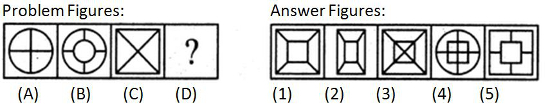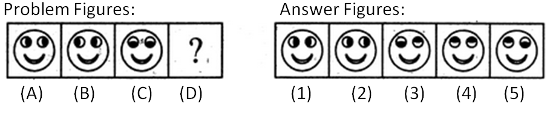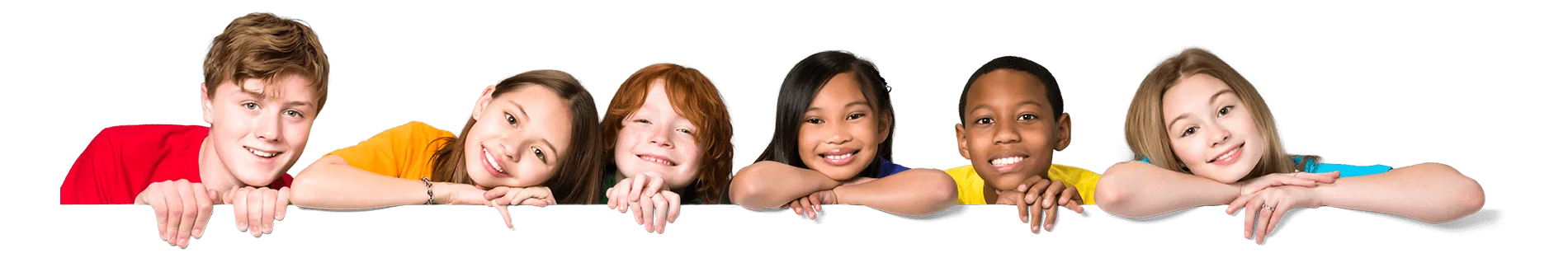Latest Results:

# 11plus Relationship sample papers

Solve this Question

#### Observe the relationship between the first two figures, and find the missing figure by applying the same relationship as that of the first two figures.1. 1 2. 2 3. 3 4. 4 5. 5

Solve this Question

#### Observe the relationship between the first two figures, and find the missing figure by applying the same relationship as that of the first two figures.1. 1 2. 2 3. 3 4. 4 5. 5

#### By observing the pattern followed by the question figures, what will be the missing figure?(consider figure A, B as one set and C, D as one set)